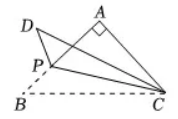$\text{A.}$ 2 或 $4-2 \sqrt{2}$ $\text{B.}$ 2 或 $4+2 \sqrt{2}$ $\text{C.}$ 4或 $4-2 \sqrt{2}$ $\text{D.}$ 4或 $4+2 \sqrt{2}$